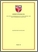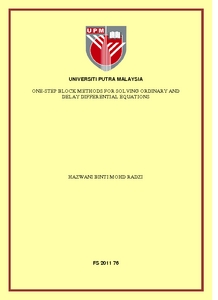# One-step block methods for solving ordinary and delay differential equations

## Citation

Mohd Radzi, Hazwani (2011) One-step block methods for solving ordinary and delay differential equations. Masters thesis, Universiti Putra Malaysia.

## Abstract

This thesis describes the implementation of one step block methods of Runge-Kutta type for solving first order Ordinary Differential Equations (ODEs) and Delay Differential Equations (DDEs). A new modified algorithm from the existing program for solving ODEs was developed. The algorithm which is based on the predictor-corrector scheme of Adams type was then extended from two up to six point one step block method. In the program, the solutions were approximated simultaneously based on equidistant block method and each of these values were calculated independently of each other. The derivation of the two point up to six point block methods based on the Newton backward divided difference formulae were presented. The stability regions were obtained and illustrated in the thesis. The numerical results showed that the developed methods are better in terms of number of total steps,failure steps and function when compared with the existing methods in most problems tested. Subsequently, the codes were then adapted to solve first order DDEs and the delay term is approximated using Neville’s interpolation. In this research, we solve DDEs of the retarded type (RDE) with single delay. The stability aspects of the one step block method when applied to DDEs were investigated and the Pand Q- stability regions were presented. In general, all the proposed methods give less number of successful steps and number of functions compared to the existing method. The numerical results have shown that the block methods proposed in this study are suitable for solving ordinary and delay differential equations.Preview
PDF
FS 2011 76R.pdfView Item